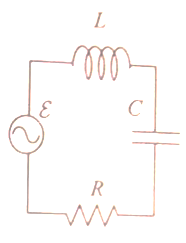Problem: An AC generator of unknown angular frequency a produces a voltage with multiple εmax. The inductance and capacitance values appear on the figure. A student measures the maximum AC voltage across each of the three circuit elements to be VL,max = 6 V, Vc,max = 3 V, and VR,max = 4V, respectively.  By what phase ∅ does the generator voltage lead the current? a. ∅  = 36.9° b. ∅  = 30.0° c. ∅ = 41.4°

FREE Expert Solution
81% (231 ratings)
Problem Details

An AC generator of unknown angular frequency a produces a voltage with multiple εmax. The inductance and capacitance values appear on the figure. A student measures the maximum AC voltage across each of the three circuit elements to be VL,max = 6 V, Vc,max = 3 V, and VR,max = 4V, respectively.  By what phase ∅ does the generator voltage lead the current?

a. ∅  = 36.9°

b. ∅  = 30.0°

c. ∅ = 41.4°